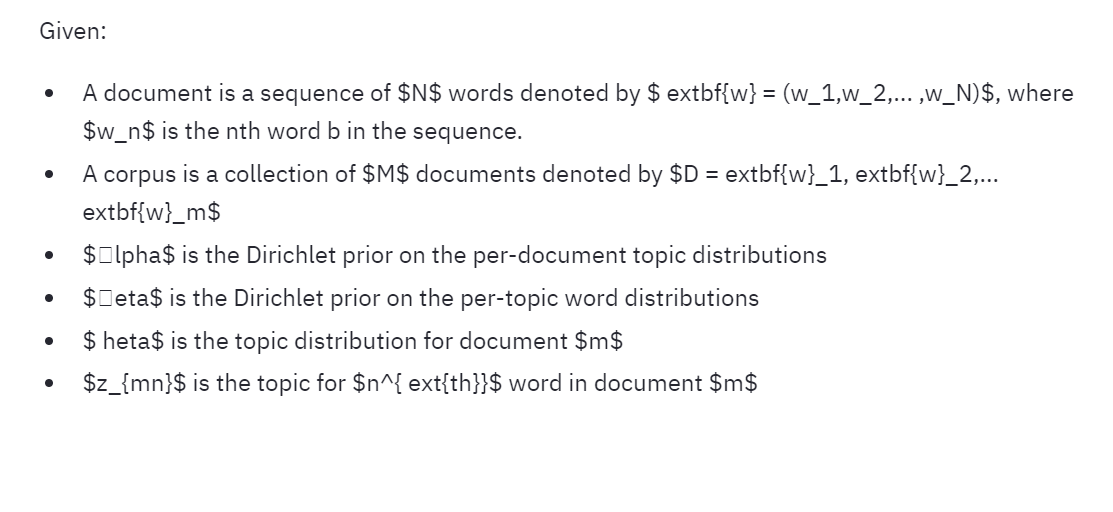# Request: Support for LaTeX in Streamlit markdown #296

Closed
opened this issue Oct 7, 2019 · 3 comments
Closed

# Request: Support for LaTeX in Streamlit markdown#296

opened this issue Oct 7, 2019 · 3 comments
Labels

### Problem

Some users community members have asked for Latex support:

### Solution

According to veered support for Latex via MathJax really ought to be built into Streamlit. It’s super helpful!

This sounds right to me, and a little research suggests it's possible! :)changed the title Request: Support for Latex in Streamlit markdown Request: Support for LaTeX in Streamlit markdown Oct 15, 2019
Merged

###lyqht commented Dec 9, 2019 • edited

 Hi, i'm not able to get latex to render even after upgrading to v 50.2! [streamlit ver 48.2]with this code st.write(""" Given: - A document is a sequence of $N$ words denoted by $\textbf{w} = (w_1,w_2,... ,w_N)$, where $w_n$ is the nth word b in the sequence. - A corpus is a collection of $M$ documents denoted by $D = \textbf{w}_1, \textbf{w}_2,...\textbf{w}_m$ - $\alpha$ is the Dirichlet prior on the per-document topic distributions - $\beta$ is the Dirichlet prior on the per-topic word distributions - $\theta$ is the topic distribution for document $m$ - $z_{mn}$ is the topic for $n^{\text{th}}$ word in document $m$ """) 

###arraydude commented Dec 9, 2019

 Hey @lyqht , The string needs to be "raw" because of the backslashes, please try this: st.write(r""" Given: - A document is a sequence of $N$ words denoted by $\textbf{w} = (w_1,w_2,... ,w_N)$, where $w_n$ is the nth word b in the sequence. - A corpus is a collection of $M$ documents denoted by $D = \textbf{w}_1, \textbf{w}_2,...\textbf{w}_m$ - $\alpha$ is the Dirichlet prior on the per-document topic distributions - $\beta$ is the Dirichlet prior on the per-topic word distributions - $\Theta$ is the topic distribution for document $m$ - $z_{mn}$ is the topic for $n^{\text{th}}$ word in document $m$ """)